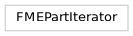# fmeobjects.FMEPartIteratorGet the part's dimension. Get all the part's coordinates in a list. Get the coordinate at the specified index. Get the number of vertices.
class FMEPartIterator

FME Part Iterator Class

init()

`FMEPartIterator` should not be constructed directly. Instead, use the iterator semantics of `FMEFeature` to get an `FMEPartIterator` which can be used to iterate over its parts.

__init__(*args, **kwargs)
dimension()

Get the part’s dimension. Returns either `FME_TWO_D` or `FME_THREE_D`.

Return type:

int

getAllCoordinates()

Get all the part’s coordinates in a list. Each coordinate is a tuple. All returned tuples have the same dimension as the part.

Return type:

list[tuple[float]]

Returns:

A list of the part’s coordinates.

getCoordinate(index)

Get the coordinate at the specified index. The dimension of the tuple equals the dimension of the part.

Parameters:

index (int) – The index at which the coordinate is retrieved.

Return type:
Returns:

The coordinate at the given index.

numVertices()

Get the number of vertices.

Return type:

int